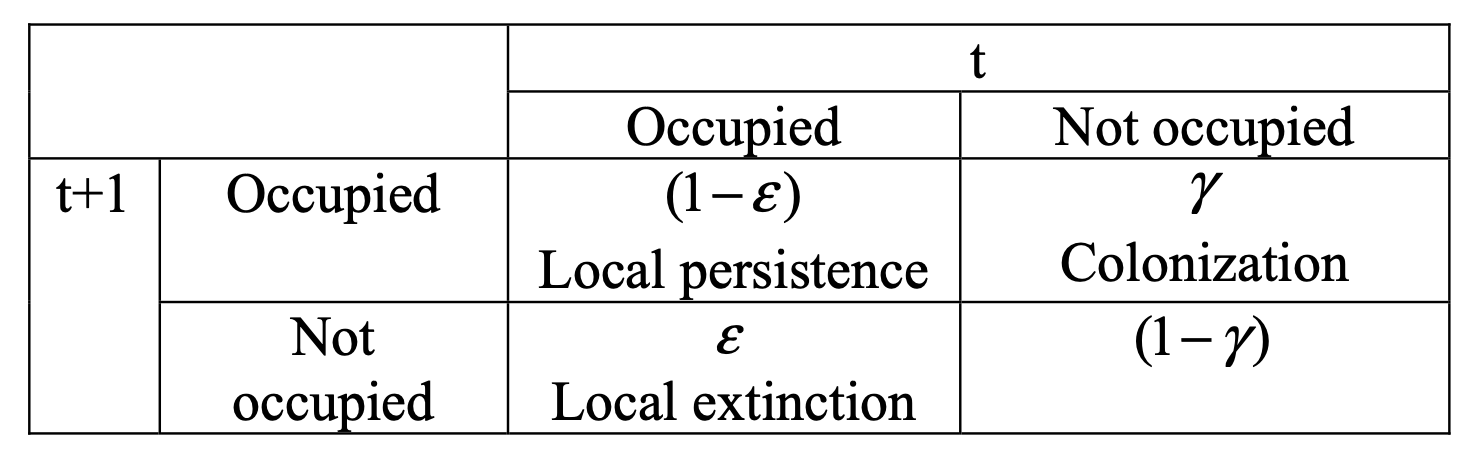This week’s homework provides you with an introduction to modeling occupancy data collected for a single-species across multiple seasons. You’ll work with data collected on northern spotted owls (Strix occidentalis caurina) and reported on by MacKenzie et al. (2003).

Background

You will conduct modeling that will show you some of the important ways that you can examine changes in occupancy and assess equilibrium with this model type. The data set consists of detection/non-detection data for northern spotted owls on multiple sites in multiple seasons (first year = 1997). We will model rates of (1) occupancy, (2) detection, (3) colonization, and (4) extinction. Here, we’ll use the parameters $$\psi$$ for occupancy rate, $$p$$ for detection rate, $$\gamma$$ for colonization rate (going from un-occupied to occupied from 1 year to the next), and $$\epsilon$$ for the local extinction rate (from 1 year to the next). Given this, $$1-\epsilon$$ is the rate at which occupied sites remain occupied, and $$1-\gamma$$ is the rate at which un-occupied sites remain un-occupied. Given the background you have in robust design, you should be able to see that the probability that a site is occupied at time $$t+1$$ may or may not depend on its occupancy status in time $$t$$, i.e., it can be either 1st-order Markovian $$(\gamma \ne 1-\epsilon)$$ or random $$(\gamma = 1-\epsilon)$$.Set up a new analysis in Program MARK using “Robust Design Occupancy” as the data type. After you do this, a new window will open that allows you to choose which parameterization to use for the modeling. We’ll start with a parameterization that works with initial occupancy ($$\psi_{1997}$$), $$\gamma_t$$, $$\epsilon_t$$, and $$p_{session, t}$$.